This page has been robot translated, sorry for typos if any. Original content here.

# § 8 Counter constant.

In the passports for electric meters, such a parameter as the counter constant is indicated. This section describes what it is and what it is for.

It was said above that the moment of rotation of the counter disk is proportional to the load, i.e.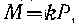,
and the opposing moment is proportional to the frequency of rotation of the disk, i.e.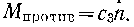If the disk rotated for t units of time, then at equilibrium (i.e., at a steady speed) we will have.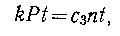and since Pt = A and nt = N , then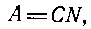where N is the number of revolutions of the disk corresponding to energy A; C is the counter constant.

The last expression shows that the amount of energy measured by the counter is proportional to the number of revolutions of the disk. The disk with the help of a worm pair is connected to a counting mechanism, which determines the amount of electricity. Unlike pointer indicating devices, in which the measured value is determined by the reading of the arrow, when measuring energy, the meter must make two counts: A1 at time t1 and A2 at time t2. Then the measured amount of energy A for a period of time t = t2-t1 is determined as the difference between the two readings, i.e.
A = A2-A1
The counter constant is determined from the expression
C = A / N.

The constant indicates the number of units of energy per disk revolution. It must be borne in mind that the nominal counter constant With nom is determined by the readings of the counting mechanism for one revolution of the disk and is unchanged. The actual counter constant C is determined by the actual amount of energy taken into account by the counter per disk revolution.
Then the error of the counter can be expressed: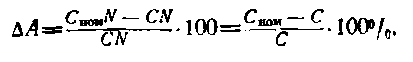The number of revolutions of the disk, during which the readings of the counting mechanism are changed per unit of the measured value, for example, 1 kW * h, is called the gear ratio of the counter. This counter parameter is also an invariable purely mechanical quantity and is associated with the gear ratio of the counting mechanism.
The counter constant and the gear ratio of the counter are interconnected. It is customary to define the counter constant as the number of watts per second per disk revolution. If the gear ratio is N A rev / kW * h, then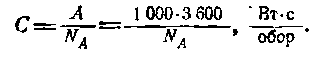The gear ratio of the meter is usually indicated on the meter: 1 kW * h = N A rpm. For example, if 1 kWh = 1250 rpm of the disk is indicated on the flap, then the counter constant will be equal to: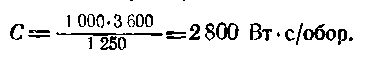At rated load, the disk rotates at a certain frequency, called rated. This parameter is sometimes indicated in the technical specification of the meter (rounded revolutions per minute). The nominal speed indirectly characterizes the wear resistance of the counter disc mounts: the smaller it is, the more durable the mounts are.